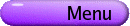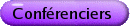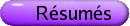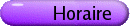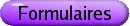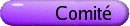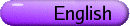Problèmes d'exploration de salle de classe en calcul
Org: Peter Taylor (Queen's)
[PDF]

SERGE D'ALESSIO AND IAN VANDERBURGH, Faculty of Mathematics, University of Waterloo
Chasing Imaginary Triangles
[PDF]

This presentation concerns right-angled triangles. In particular, we are interested in computing the length of its hypotenuse (h) given the triangle's perimeter (P) and area (A). One method of determining h involves the formula:

 h = P2 - 4A 2P .
However, as we will demonstrate, this formula can lead to an incorrect result. To resolve this a condition between A and P was derived that dictates when the above formula can be used. Other interesting issues surrounding right-angled triangles will also be explored along the way.

RICHARD HOSHINO, Dalhousie University, Department of Mathematics, Halifax, NS B3H 3J5
A Heart-Stopping Solution
[PDF]

During a first-year calculus class I taught several years ago, my midterm took place on Valentine's Day. To fit the occassion, a problem on the exam asked the students to determine the polar equation of a heart. Overall, the students found the question to be very challenging, as we had only discussed how to sketch a curve given the polar equation; and this question required them to go backwards. Some of my students came up with the correct answer I was looking for, namely r = 4 - 4sinq, where q runs from 0 to 2P. However, one student came up with a completely different equation, using various transformations and translations of the absolute value function. In this brief session, we will determine "Steve's equation", and unpack the sophisticated ideas involved in his solution. We hope to present this heart-stopper as an example of a beautiful and pedagogically-rich problem in the first-year calculus course.

VED MADAN, St. Mary's University College, 14500 Bannister Rd. SE, Calgary, Alberta T2X 1Z4
Information Technology-Impact on Calculus Problem Solving Skills/Historical Perspective:
[PDF]

Changes in mathematics instructions over the past three decades have been necessitated by increasing student numbers at post-secondary institutions. Advances in technology have benefited mathematics instruction, particularly in the area of calculus due to its analytic, graphic and numeric approaches to solving real life problems.

Until the 1970s, Calculus was taught with traditional text books. In the 1980s, non-linear processes in Reform Calculus involved the use of graphing calculators and computer spreadsheets. Websites designed to promote the sharing of information and ideas became popular in the 1990s. In the 21st century, increasingly sophisticated technological tools such as teleconferencing and chat rooms are enhancing calculus problem solving skills with one-to-many and many-to-many web-based collaborative instructional strategies for student-centered learning.

The pitfalls of advances in technology also need to be recognized. For example, students today may be lacking basic skills that would allow them to perform calculations without the benefit of calculator.

This presentation will review some major developments in technology since the 1970s and share information on how technology advancement has influenced calculus problem solving skills-both positively and negatively.

PETER TAYLOR, Queen's University, Kingston, ON K7L 3N6
Introduction and some "Executive Class" Examples
[PDF]

I will briefly indicate a number of examples of exploratory calculus problems we have used in our special MATH 121 "Executive Class". I'm hoping that these example will spawn further ideas and reflections in the workshop activity to come at the end of both the morning and the afternoon sessions. Others are invited to contribute informally at that time.